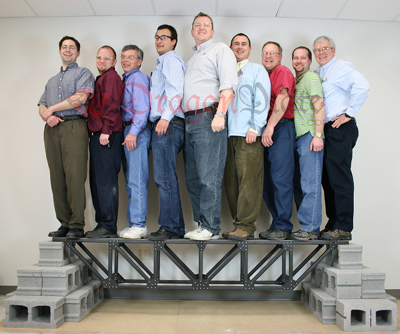# Just How Strong is Carbon Fiber?The most valuable characteristics of carbon fiber are its strength, stiffness, and light weight. Carbon fiber is made of very fine, crystalline filaments of carbon in “tows”, which contain thousands of individual filaments. These yarn-like strands are woven together into cloth of various weaves. When layers of carbon fiber cloth are bonded together, into a carbon fiber plate or sheet, the carbon fiber laminate is exceedingly strong. So how strong is it?

## Classifying Carbon Fiber Stiffness and Strength

Tensile modulus is defined as “the ratio of stress (force per unit area) along an axis to strain (ratio of deformation over initial length) along that axis.” Also known as the stiffness, tensile modulus can predict the elongation or compression of material as long as the stress is lower than the tensile strength of the material.

Carbon fibers are classified based on the tensile modulus of the fibers. The English unit of measure is pounds of force per square inch of cross-sectional area, abbreviated as psi (or ksi for thousand psi and MSI for million psi) . There are five carbon fiber classifications: low modulus, standard modulus, intermediate modulus, high modulus, and ultrahigh modulus.

 Carbon Fiber Class Low Modulus Standard Modulus Intermediate Modulus High Modulus Ultrahigh Modulus Tensile Modulus GPa <227 227 289 393 758 Tensile Modulus MSI <33 33 42 57 110

The stiffness and strength of a laminated carbon fiber epoxy sheet or plate are determined by:

• The material properties of the carbon fiber
• The layup schedule of the fibers (fiber orientation, weave type and thickness of laminate plies)
• The fiber/resin ratio

A good estimate for tensile modulus for a balanced, symmetrical, 0/90deg layup schedule would be 10 MSi (70 GPA). A good estimate for tensile strength for the same layup would be 87 KSI (600 MPA).

By comparison, steel has a tensile modulus of around 29 MSI (200 GPa) and a tensile strength of 61 KSI (420 MPA ). The tensile modulus of aluminum is about 10 MSI (69 GPa) and tensile strength is 40 KSI (276 MPA)

As can be seen from the table below carbon fiber laminate has the highest specific Tensile Strength and the highest Specific Stiffness

 Material Tensile Modulus GPa Tensile Strength MPa Density g/cm3 Specific Tensile StrengthPa m3/kg Specific Tensile ModulusMPa m3/kg Aluminum 69 276 2.7 102 25.5 Steel 200 420 7.9 53 25.3 Carbon Fiber Laminate 70 600 1.5 400 46.7

Ultra high modulus carbon fibers are approximately 3 times stiffer than standard modulus but are not as strong. In addition, carbon fiber has superior fatigue resistance properties when compared to both steel and aluminum. Also, when combined with appropriate resins, carbon fibers are among the most corrosion-resistant materials on the market.

## How Does Carbon Fiber Compare to Other Materials?

Fiberglass and Kevlar® laminates have densities close to that of carbon fiber. However, carbon fiber is stronger and much stiffer than either fiberglass or Kevlar®

Comparing characteristics of carbon fiber to other materials is not always straightforward. There are multiple ways to measure strength, and this can make it difficult to make objective comparisons. However, when looking strictly at specific tensile modulus and specific tensile strength the choice is obviousStrength isn’t a top priority for every project. Often other factors like flexibility or manufacturing requirements must be considered when selecting materials for a project. When strength and stiffness and weight are paramount, carbon fiber is typically the best choice.

 Material Modulus of Elasticity GPa Tensile Strength MPa Density g/cm3 Specific Tensile Strength Pa m3/kg Specific Tensile Modulus MPa m3/kg Fiberglass 25 440 1.9 231 13.2 Kevlar® (Aramid) 30 480 1.4 333 20.8 Carbon Fiber 70 600 1.5 400 46.7

Comparing characteristics of carbon fiber to other materials is not always straightforward. There are multiple ways to measure strength, and this can make it difficult to make objective comparisons. However, when looking strictly at specific tensile modulus and specific tensile strength the choice is obvious

Strength isn’t a top priority for every project. Often other factors like flexibility or manufacturing requirements must be considered when selecting materials for a project. When strength and stiffness and weight are paramount, carbon fiber is typically the best choice.